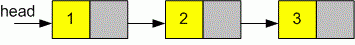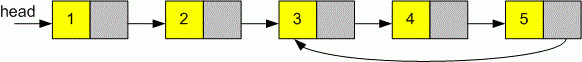Competitions

Given a linked list. Find the distance from the head of the list to the node where cycle starts. If there is no cycle, return -1.

Definition of a single linked list:

// Java
class ListNode {
int val;
ListNode next;
ListNode(int x) {
val = x;
next = null;
}
}
// C++
class ListNode
{
public:
int val;
ListNode *next;
ListNode(int x) : val(x), next(NULL) {}
};
// C
struct ListNode
{
int val;
struct ListNode *next;
};

Implement a function DistanceToCycle that returns the distance from the head of the list to the node where cycle starts. If there is no cycle, return -1.

// Java
// C, C++

ExampleFunction DistanceToCycle returns -1 because this linked list does not contain a cycle.Function DistanceToCycle returns 2 - the distance from the head of the list to the node where cycle starts. (123).

Time limit 1 second
Memory limit 128 MiB
Author Michael Medvediev